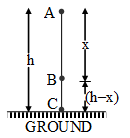Ex﻿

# 3. Work and Energy

#### Inter conversion of potential And kinetic energy

Mechanical Energy of a Freely Falling Body :
Assume, a body of mass m is at rest at a height h from the earth's surface, as it starts falling, its velocity after travelling a distance x (point B) becomes v and its velocity on the earth's surface is v'.
Mechanical energy of the body at point A :
EA = Kinetic energy + Potential energy
EA = m(0)2 + mgh
EA = mgh ………(i)

Mechanical energy of the body at point B :
EB =mv2 + mg (h – x) ……. ..(ii)Mechanical energy of the body at point C :
EC =m (v')2 + mg × 0
EC =m (v')2 ……..(iv)
Use : EA = EB = EC
Hence, when a body falls freely, its mechanical energy will be constant. That means, the total energy of the body during free fall, remains constant at all positions. However, the form of energy keeps on changing at all points during the motion.

If you want to give information about online courses to other students, then share it with more and more on Facebook, Twitter, Google Plus. The more the shares will be, the more students will benefit. The share buttons are given below for your convenience.
×

#### NTSE Physics (Class X)

• Electricity
• Magnetic Effect of Curent
• Light Reflection
• Light Refraction
• Human Eye & Colourful World
• Source of Energy
• Motion

#### NTSE Physics (Class IX)

• Force & laws of motion
• Work and Energy
• Gravitation
• Sound

SHOW CHAPTERS